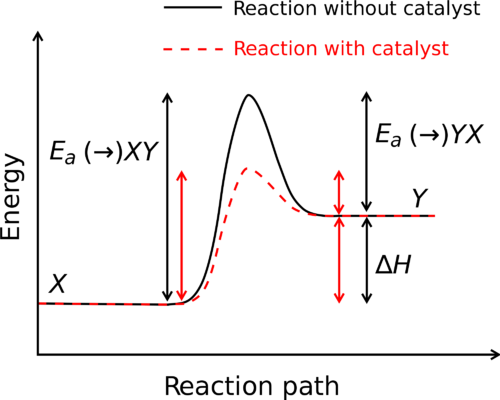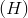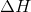# Enthalpy

• Define enthalpy.
• List factors that influence enthalpy.Energy changes in a chemical reaction.  Ea means “Energy of Activation” and represents the energy required to get the reaction going. Does the catalyst affect enthalpy?

The factors influencing a reaction are complicated and varied.  Since a catalyst affects activation energy, we might assume it would have some sort of impact on enthalpy, but it does not. The change in enthalpy of a reaction depends solely on the chemical compositions of the reactants and products, not on the path taken to get from one to the other.

A chemical reaction takes place when the molecules of the reactants collide with each other, are oriented in just the right way and have enough energy to collide.  All three things must be true in order for a reaction to take place.  So even if the reactants are oriented in the correct way, the amount of energy needed to break the bonds between the atoms of the reactants (the activation energy, Ea in the figure above) must be sufficient.  Whenever the energy in the collision is less than the activation energy, the molecules of the reactants may come in contact with each other but no reaction will occur.  A catalyst can lower the activation energy (Ea) of a reaction but the catalyst does not affect the overall enthalpy of the reaction.

### Enthalpy

Heat changes in chemical reactions are most often measured in the laboratory under conditions in which the reacting system is open to the atmosphere. In that case, the system is at a constant pressure. Enthalpyis the heat content of a system at constant pressure. Chemists routinely measure changes in enthalpy of chemical systems as reactants are converted into products. The heat that is absorbed or released by a reaction at constant pressure is the same as the enthalpy change, and is given the symbol. Unless otherwise specified, all reactions in this material are assumed to take place at constant pressure.

The change in enthalpy of a reaction is a measure of the differences in enthalpy of the reactants and products.  The change in enthalpy is also called the heat of the reaction and given the symbol ΔH.  The heat of a reaction is the difference between the energy of bond formation (in the products) and bond breaking (in the reactants). ΔH can be negative or positive depending on whether the reaction is exothermic (heat is released, negative sign, -ΔH) or endothermic (heat is absorbed, positive sign, +ΔH).

ΔH = Hproducts – H reactants

The enthalpy of a system is determined by the energies needed to break chemical bonds and the energies needed to form chemical bonds. Energy needs to be put into the system in order to break chemical bonds – they do not come apart spontaneously in most cases. Bond formation to produce products will involve release of energy. The change in enthalpy shows the trade-offs made in these two processes. Does it take more energy to break bonds that that needed to form bonds? If so, the reaction is endothermic and the enthalpy change is positive. If more energy is produced in bond formation than that needed for bond breaking, the reaction is exothermic and the enthalpy is negative.

Several factors influence the enthalpy of a system. Enthalpy is an extensive property, determined in part by the amount of material we work with. The state of reactants and products (solid, liquid, or gas) influences the enthalpy value for a system. The direction of the reaction affects the enthalpy value. A reaction that takes place in the opposite direction has the same numerical enthalpy value, but the opposite sign.

#### Summary

• Enthalpy is related to the heat of a reaction.
• Factors influencing entropy are described.

#### Practice

Questions

Read the material at the link below and answer the following questions:

http://www.ausetute.com.au/heatreact.html

1. What is the heat of reaction?
2. What units are used?
3. During the experimental measurement of heat of reaction, is heat lost to the surroundings or gained from the surroundings?

#### Review

Questions

1. What is enthalpy?
2. What is an extensive property?
3. Do the states of reactants and products influence enthalpy values?
• enthalpy: The heat content of a system at constant pressure.
• activation energy (Ea): the amount of energy needed to break the bonds between the atoms of the reactants
• heat of reaction: The difference between the energy of bond formation (in the products) and bond breaking (in the reactants); also the change in enthalpy during a reaction